# Integral extension implies surjective map on spectra

This article gives the statement, and possibly proof, of a fact about how a property of a homomorphism of commutative unital rings, forces a property for the induced map on spectra
View other facts about induced maps on spectra

## Name

This result is sometimes termed lying over, and is a precursor to the going up theorem.

## Statement

Suppose$S$ is an integral extension of a ring$R$, in other words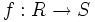$f:R \to S$ is an injective homomorphism of commutative unital rings with the property that every element of$S$ is integral over the image of$R$. Then, the following are true.

The map: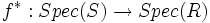$f^*: Spec(S) \to Spec(R)$

from the spectrum of$S$ to that of$R$, that sends a prime ideal of$S$ to its contraction in$R$, is surjective. In other words, every prime ideal of$R$ occurs as the contraction of a prime ideal of$S$.

This result is sometimes termed the lying over theorem.

Note that injectivity of$f$ is crucial for surjectivity of the map on spectra; this is analogous to the fact that surjective ring homomorphisms induce injective maps on spectra.

## Proof

The goal is to prove that starting with a prime ideal$P$ of$R$, we can find a prime ideal$Q$ of$S$ such that$f^{-1}(Q) = P$.

We localize$R$ at$P$, and localize$S$ at the image of$U = R \setminus P$ to get$S'$. Then$R_P$ is a local ring with unique maximal ideal$P' = PR_P$, and$f$ induces a map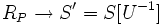$R_P \to S' = S[U^{-1}]$.

We thus have an inclusion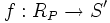$f:R_P \to S'$. Consider the image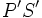$P'S'$. This is an ideal of$S'$. If$P'S'$ is a proper ideal, it is contained in some maximal ideal$M$, and the contraction of that maximal ideal to$R_P$ is precisely$P'$. Contracting back along the localization, we find a prime ideal of$S'$, whose contraction is exactly$P$. (we are using the fact that contracting a maximal ideal of$S'$ yields a prime, though not necessarily maximal, ideal of$S$).

Thus, the main goal is to show that$P'S' \ne S'$ (this is where we need to use integrality). The idea is to construct a$R$-subalgebra of$S'$, called$S''$, that is finite over$R_P$, and use Nakayama's lemma to derive a contradiction. Here are the steps:

• Since$S$ is integral over$R$,$S'$ is integral over$R_P$
• If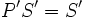$P'S' = S'$, then the element$1 \in S'$ can be written as a$P'$-linear combination of finitely many elements from$S'$
• Let$S''$ be the$R_P$-subalgebra generated by these finitely many elements. Then$S''$ is finitely generated and integral over$R_P$, hence it is finitely generated as a module over$R_P$. For full proof, refer: finitely generated and integral implies finite
• We thus have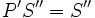$P'S'' = S''$ (since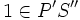$1 \in P'S''$). Since$P'$ is the Jacobson radical of$R_P$, Nakayama's lemma tells us that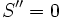$S'' = 0$, yielding a contradiction.